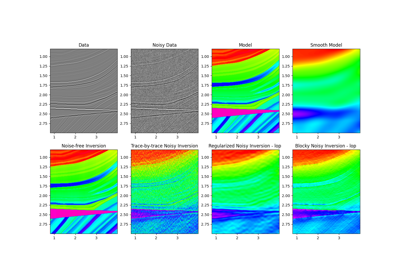# pylops.avo.poststack.PoststackLinearModelling¶

pylops.avo.poststack.PoststackLinearModelling(wav, nt0, spatdims=None, explicit=False, sparse=False)[source]

Post-stack linearized seismic modelling operator.

Create operator to be applied to an elastic parameter trace (or stack of traces) for generation of band-limited seismic post-stack data. The input model and data have shape $$[n_{t0} (\times n_x \times n_y)]$$.

Parameters: wav : np.ndarray Wavelet in time domain (must have odd number of elements and centered to zero). If 1d, assume stationary wavelet for the entire time axis. If 2d, use as non-stationary wavelet (user must provide one wavelet per time sample in an array of size $$[n_{t0} \times n_{wav}]$$ where $$n_{wav}$$ is the length of each wavelet). Note that the dtype of this variable will define that of the operator nt0 : int Number of samples along time axis spatdims : int or tuple, optional Number of samples along spatial axis (or axes) (None if only one dimension is available) explicit : bool, optional Create a chained linear operator (False, preferred for large data) or a MatrixMult linear operator with dense matrix (True, preferred for small data) sparse : bool, optional Create a sparse matrix (True) or dense (False) when explicit=True Pop : LinearOperator post-stack modelling operator. ValueError If wav is two dimensional but does not contain nt0 wavelets

Notes

Post-stack seismic modelling is the process of constructing seismic post-stack data from a profile of an elastic parameter of choice in time (or depth) domain. This can be easily achieved using the following forward model:

$d(t, \theta=0) = w(t) * \frac{dln(m(t))}{dt}$

where $$m(t)$$ is the elastic parameter profile and $$w(t)$$ is the time domain seismic wavelet. In compact form:

$\mathbf{d}= \mathbf{W} \mathbf{D} \mathbf{m}$

In the special case of acoustic impedance ($$m(t)=AI(t)$$), the modelling operator can be used to create zero-offset data:

$d(t, \theta=0) = \frac{1}{2} w(t) * \frac{dln(m(t))}{dt}$

where the scaling factor $$\frac{1}{2}$$ can be easily included in the wavelet.

## Examples using pylops.avo.poststack.PoststackLinearModelling¶07. Post-stack inversion